# Python 图像处理 OpenCV （15）：图像轮廓

15 篇文章 7 订阅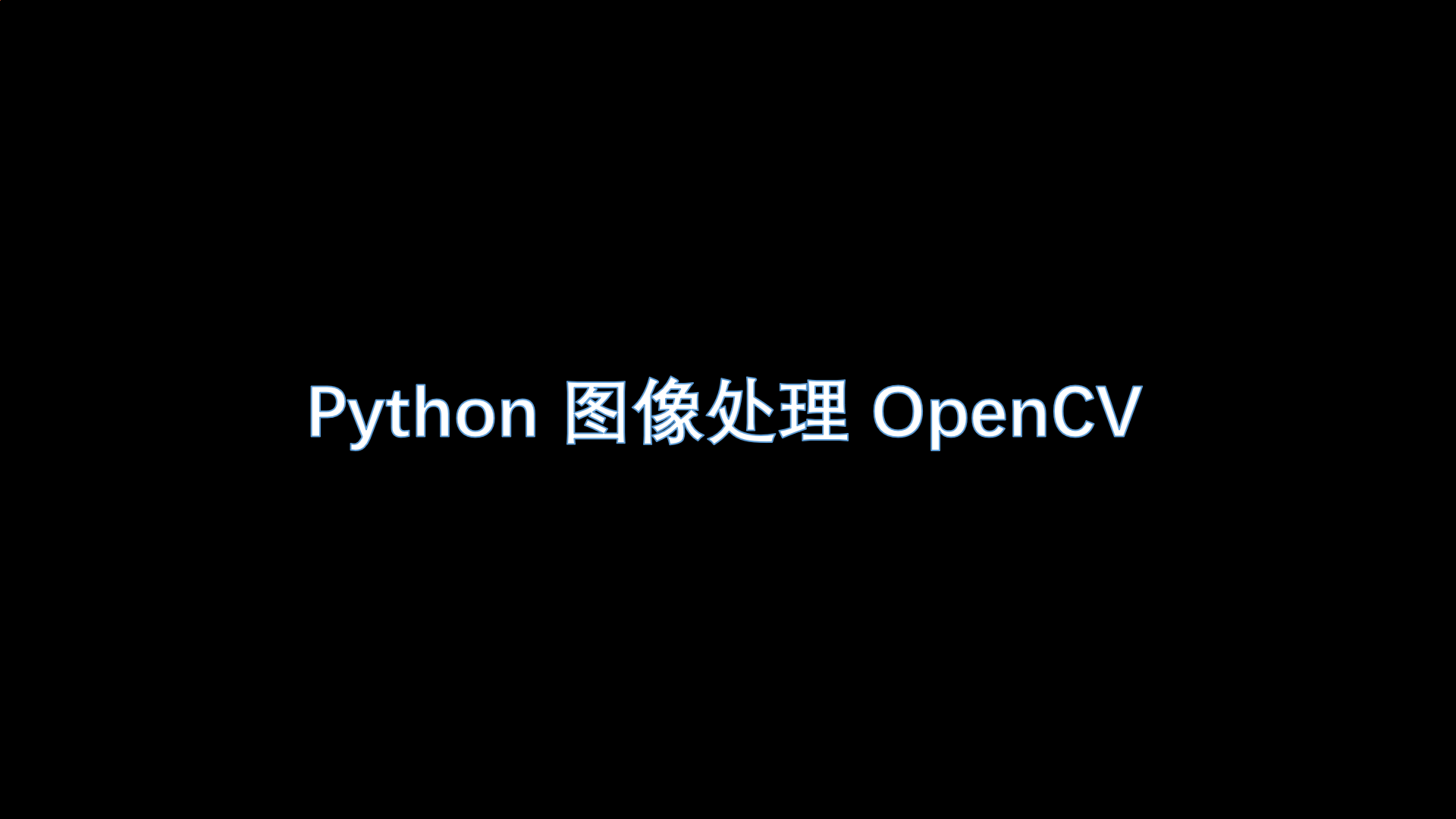「Python 图像处理 OpenCV （1）：入门」

「Python 图像处理 OpenCV （2）：像素处理与 Numpy 操作以及 Matplotlib 显示图像」

「Python 图像处理 OpenCV （3）：图像属性、图像感兴趣 ROI 区域及通道处理」

「Python 图像处理 OpenCV （4）：图像算数运算以及修改颜色空间」

「Python 图像处理 OpenCV （5）：图像的几何变换」

「Python 图像处理 OpenCV （6）：图像的阈值处理」

「Python 图像处理 OpenCV （7）：图像平滑（滤波）处理」

「Python 图像处理 OpenCV （8）：图像腐蚀与图像膨胀」

「Python 图像处理 OpenCV （9）：图像处理形态学开运算、闭运算以及梯度运算」

「Python 图像处理 OpenCV （10）：图像处理形态学之顶帽运算与黑帽运算」

「Python 图像处理 OpenCV （11）：Canny 算子边缘检测技术」

「Python 图像处理 OpenCV （12）： Roberts 算子、 Prewitt 算子、 Sobel 算子和 Laplacian 算子边缘检测技术」

「Python 图像处理 OpenCV （13）： Scharr 算子和 LOG 算子边缘检测技术」

「Python 图像处理 OpenCV （14）：图像金字塔」

## 引言

Q：什么是轮廓？

A：轮廓是一系列相连的点组成的曲线，代表了物体的基本外形，相对于边缘，轮廓是连续的，边缘并不全部连续。

## 寻找轮廓

Satoshi Suzuki and others. Topological structural analysis of digitized binary images by border following. Computer Vision, Graphics, and Image Processing, 30(1):32–46, 1985.

import cv2 as cv

gray_img = cv.cvtColor(img, cv.COLOR_BGR2GRAY)
# 降噪
ret, thresh = cv.threshold(gray_img, 127, 255, 0)
# 寻找轮廓
contours, hierarchy = cv.findContours(thresh, cv.RETR_TREE, cv.CHAIN_APPROX_NONE)

print(len(contours))


retval, dst = cv.threshold(src, thresh, maxval, type[, dst] )

• dst：结果图像。
• src：原图像。
• thresh：当前阈值。
• maxVal：最大阈值，一般为255。
• type：阈值类型，可选值如下：
enum ThresholdTypes {
THRESH_BINARY     = 0,  # 大于阈值的部分被置为 255 ，小于部分被置为 0
THRESH_BINARY_INV = 1,  # 大于阈值部分被置为 0 ，小于部分被置为 255
THRESH_TRUNC      = 2,  # 大于阈值部分被置为 threshold ，小于部分保持原样
THRESH_TOZERO     = 3,  # 小于阈值部分被置为 0 ，大于部分保持不变
THRESH_TOZERO_INV = 4,  # 大于阈值部分被置为 0 ，小于部分保持不变
THRESH_OTSU       = 8,  # 自动处理，图像自适应二值化，常用区间 [0,255]
};


cv2.findContours(image, mode, method[, contours[, hierarchy[, offset ]]])

• image：源图像。
• mode：表示轮廓检索模式。
cv2.RETR_EXTERNAL 表示只检测外轮廓。
cv2.RETR_LIST 检测的轮廓不建立等级关系。
cv2.RETR_CCOMP 建立两个等级的轮廓，上面的一层为外边界，里面的一层为内孔的边界信息。如果内孔内还有一个连通物体，这个物体的边界也在顶层。
cv2.RETR_TREE 建立一个等级树结构的轮廓。

• method：表示轮廓近似方法。
cv2.CHAIN_APPROX_NONE 存储所有的轮廓点。
cv2.CHAIN_APPROX_SIMPLE 压缩水平方向，垂直方向，对角线方向的元素，只保留该方向的终点坐标，例如一个矩形轮廓只需4个点来保存轮廓信息。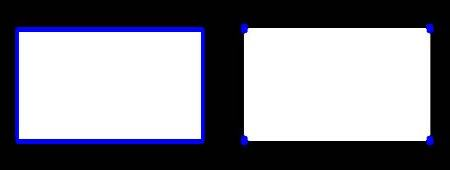## 绘制轮廓

# To draw all the contours in an image:
cv2.drawContours(img, contours, -1, (0,255,0), 3)
# To draw an individual contour, say 4th contour:
cv2.drawContours(img, contours, 3, (0,255,0), 3)
# But most of the time, below method will be useful:
cnt = contours
cv2.drawContours(img, [cnt], 0, (0,255,0), 3)


drawContours() 函数中有五个参数：

• 第一个参数是源图像。
• 第二个参数是应该包含轮廓的列表。
• 第三个参数是列表索引，用来选择要绘制的轮廓，为-1时表示绘制所有轮廓。
• 第四个参数是轮廓颜色。
• 第五个参数是轮廓线的宽度，为 -1 时表示填充。

import cv2 as cv

gray_img = cv.cvtColor(img, cv.COLOR_BGR2GRAY)
cv.imshow("img", img)
# 降噪
ret, thresh = cv.threshold(gray_img, 127, 255, 0)
# 寻找轮廓
contours, hierarchy = cv.findContours(gray_img, cv.RETR_TREE, cv.CHAIN_APPROX_NONE)

print(len(contours))

# 绘制绿色轮廓
cv.drawContours(img, contours, -1, (0,255,0), 3)

cv.imshow("draw", img)

cv.waitKey(0)
cv.destroyAllWindows()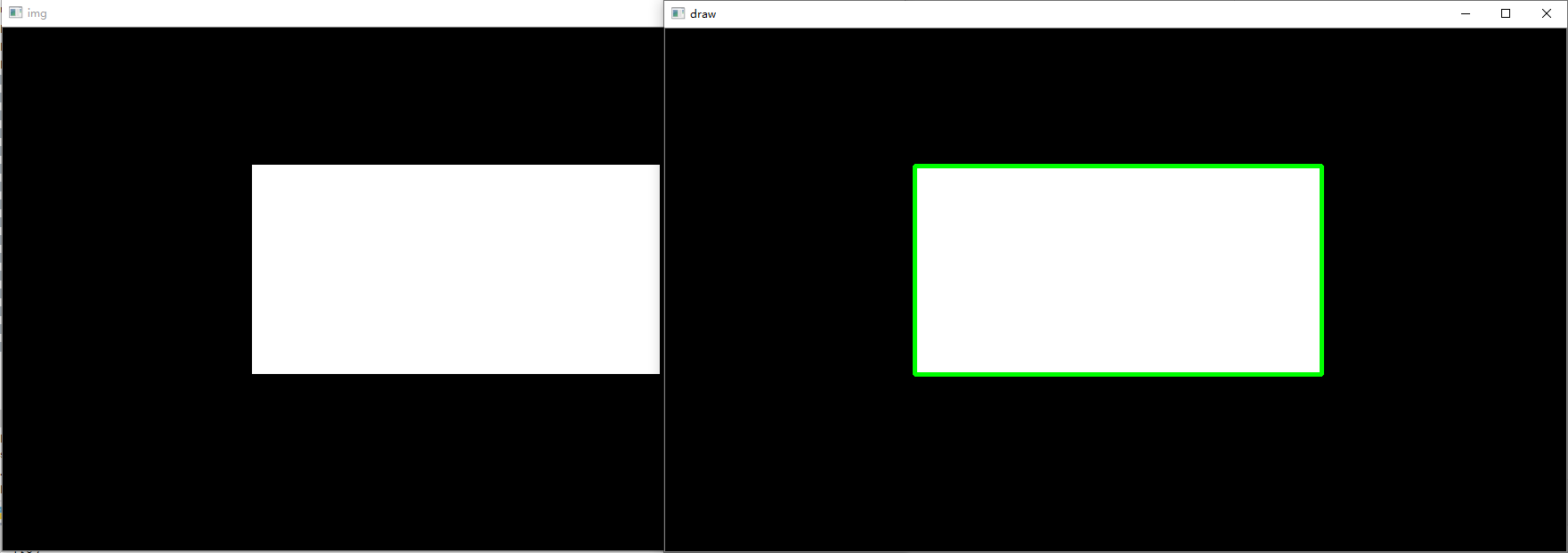## 特征矩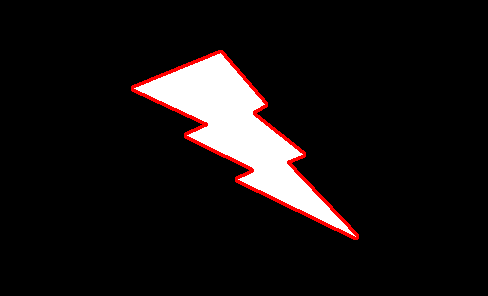moments() 函数会将计算得到的矩以字典形式返回。

import cv2 as cv

gray_img = cv.cvtColor(img, cv.COLOR_BGR2GRAY)
# 降噪
ret, thresh = cv.threshold(gray_img, 127, 255, 0)
# 寻找轮廓
contours, hierarchy = cv.findContours(gray_img, cv.RETR_TREE, cv.CHAIN_APPROX_NONE)

cnt = contours
# 获取图像矩
M = cv.moments(cnt)
print(M)

# 质心
cx = int(M['m10'] / M['m00'])
cy = int(M['m01'] / M['m00'])

print(f'质心为：[{cx}, {cy}]')


## 轮廓面积

area = cv.contourArea(cnt)
print(f'轮廓面积为:{area}')


## 轮廓周长

perimeter = cv.arcLength(cnt, True)
print(f'轮廓周长为:{perimeter}')


## 轮廓外接矩形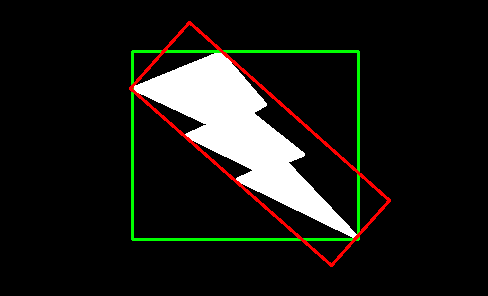import cv2 as cv
import numpy as np

gray_img = cv.cvtColor(img, cv.COLOR_BGR2GRAY)
# 降噪
ret, thresh = cv.threshold(gray_img, 127, 255, 0)
# 寻找轮廓
contours, hierarchy = cv.findContours(gray_img, cv.RETR_TREE, cv.CHAIN_APPROX_NONE)

cnt = contours

# 外接正矩形
x, y, w, h = cv.boundingRect(cnt)
cv.rectangle(img, (x, y), (x + w, y + h), (0, 255, 0), 2)

# 外接最小矩形
min_rect = cv.minAreaRect(cnt)
print(min_rect)

box = cv.boxPoints(min_rect)
box = np.int0(box)
cv.drawContours(img, [box], 0, (0, 0, 255), 2)

cv.imshow("draw", img)

cv.waitKey(0)
cv.destroyAllWindows()


boundingRect() 函数的返回值包含四个值，矩形框左上角的坐标 (x, y) 、宽度 w 和高度 h 。

minAreaRect() 函数的返回值中还包含旋转信息，返回值信息为包括中心点坐标 (x,y) ，宽高 (w, h) 和旋转角度。

## 轮廓近似

OpenCV 提供的函数是 approxPolyDP(cnt, epsilon, True) ，第二个参数 epsilon 用于轮廓近似的精度，表示原始轮廓与其近似轮廓的最大距离，值越小，近似轮廓越拟合原轮廓。第三个参数指定近似轮廓是否是闭合的。具体用法如下：

import cv2 as cv

gray_img = cv.cvtColor(img, cv.COLOR_BGR2GRAY)
# 降噪
ret, thresh = cv.threshold(gray_img, 127, 255, 0)
# 寻找轮廓
contours, hierarchy = cv.findContours(gray_img, cv.RETR_TREE, cv.CHAIN_APPROX_NONE)

cnt = contours

# 计算 epsilon ，按照周长百分比进行计算，分别取周长 1% 和 10%
epsilon_1 = 0.1 * cv.arcLength(cnt, True)
epsilon_2 = 0.01 * cv.arcLength(cnt, True)

# 进行多边形逼近
approx_1 = cv.approxPolyDP(cnt, epsilon_1, True)
approx_2 = cv.approxPolyDP(cnt, epsilon_2, True)

# 画出多边形
image_1 = cv.cvtColor(gray_img, cv.COLOR_GRAY2BGR)
image_2 = cv.cvtColor(gray_img, cv.COLOR_GRAY2BGR)

cv.polylines(image_1, [approx_1], True, (0, 0, 255), 2)
cv.polylines(image_2, [approx_2], True, (0, 0, 255), 2)

cv.imshow("image_1", image_1)
cv.imshow("image_2", image_2)
cv.waitKey(0)
cv.destroyAllWindows()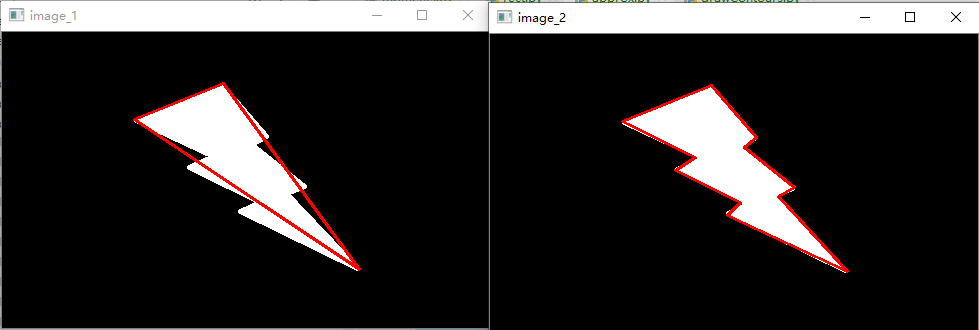## 轮廓凸包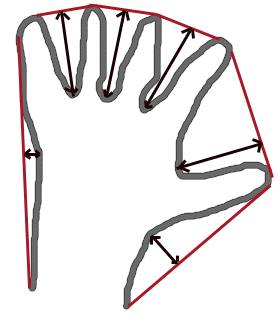import cv2 as cv

gray_img = cv.cvtColor(img, cv.COLOR_BGR2GRAY)
# 降噪
ret, thresh = cv.threshold(gray_img, 127, 255, 0)
# 寻找轮廓
contours, hierarchy = cv.findContours(gray_img, cv.RETR_TREE, cv.CHAIN_APPROX_NONE)
cnt = contours
# 绘制轮廓
image = cv.cvtColor(gray_img, cv.COLOR_GRAY2BGR)
cv.drawContours(image, contours, -1, (0, 0 , 255), 2)

# 寻找凸包，得到凸包的角点
hull = cv.convexHull(cnt)

# 绘制凸包
cv.polylines(image, [hull], True, (0, 255, 0), 2)

cv.imshow("image", image)
cv.waitKey(0)
cv.destroyAllWindows()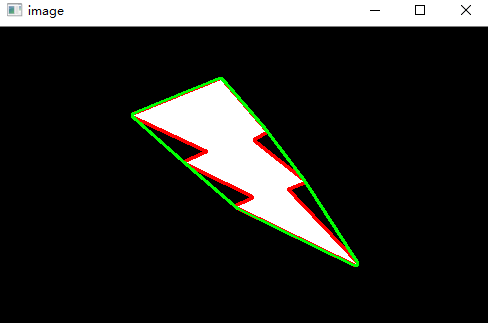print(cv.isContourConvex(hull)) # True


## 最小闭合圈

import cv2 as cv

gray_img = cv.cvtColor(img, cv.COLOR_BGR2GRAY)
# 降噪
ret, thresh = cv.threshold(gray_img, 127, 255, 0)
# 寻找轮廓
contours, hierarchy = cv.findContours(gray_img, cv.RETR_TREE, cv.CHAIN_APPROX_NONE)
cnt = contours

# 绘制最小外接圆
center = (int(x), int(y))
cv.circle(img, center, radius, (0, 255, 0), 2)

cv.imshow("img", img)
cv.waitKey(0)
cv.destroyAllWindows()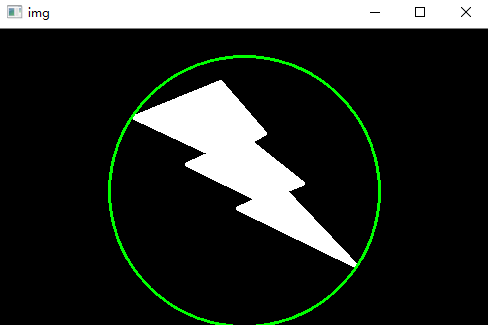ellipse = cv.fitEllipse(cnt)
cv.ellipse(img, ellipse, (0, 255, 0), 2)## 参考

https://zhuanlan.zhihu.com/p/61328775

https://zhuanlan.zhihu.com/p/7778334708-0421
08-111万+06-1729
04-283714
10-229842
05-29141
01-044万+
04-25118
06-081万+
08-291632
01-082万+
11-29483
09-08130
12-08432
12-08400
05-267516
05-03515
08-151431点击重新获取扫码支付余额充值